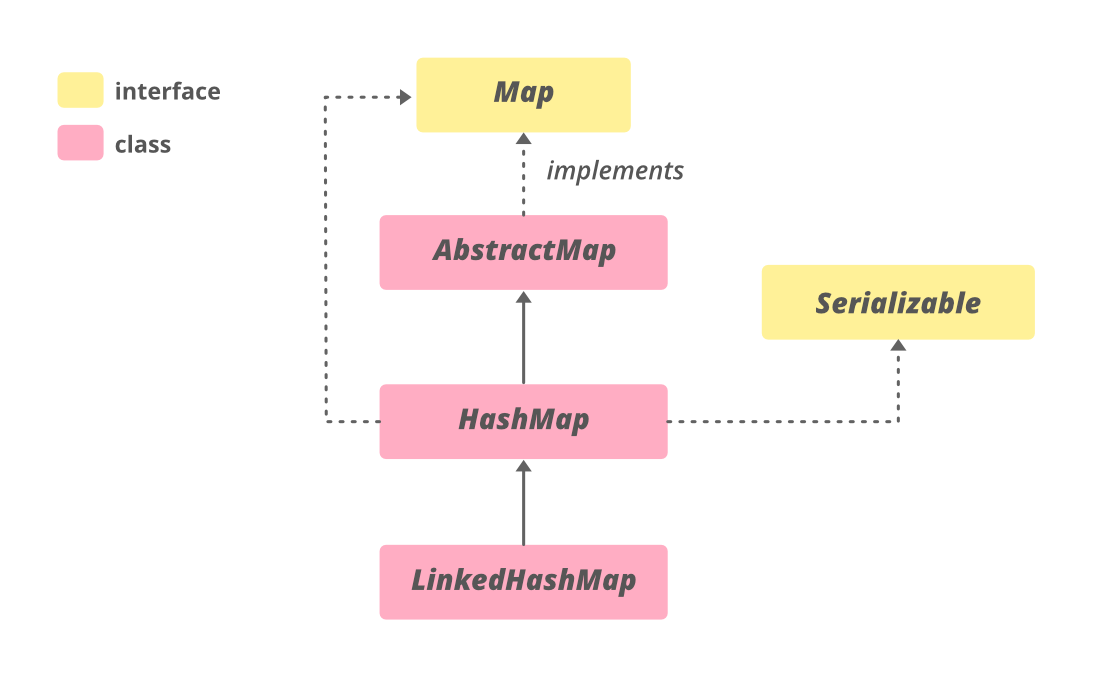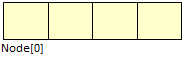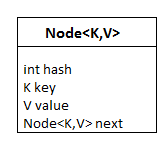Related Articles

# HashMap in Java with Examples

• Difficulty Level : Medium
• Last Updated : 20 Jul, 2021

HashMap<K, V> is a part of Java’s collection since Java 1.2. This class is found in java.util package. It provides the basic implementation of the Map interface of Java. It stores the data in (Key, Value) pairs, and you can access them by an index of another type (e.g. an Integer). One object is used as a key (index) to another object (value). If you try to insert the duplicate key, it will replace the element of the corresponding key.

HashMap is similar to HashTable, but it is unsynchronized. It allows to store the null keys as well, but there should be only one null key object and there can be any number of null values.  This class makes no guarantees as to the order of the map. To use this class and its methods, you need to import java.util.HashMap package or its superclass.

## Java

 `// Java program to illustrate HashMap class of java.util``// package` `// Importing HashMap class``import` `java.util.HashMap;` `// Main class``public` `class` `GFG {` `    ``// Main driver method``    ``public` `static` `void` `main(String[] args)``    ``{``        ``// Create an empty hash map by declaring object``        ``// of string and integer type``        ``HashMap map = ``new` `HashMap<>();` `        ``// Adding elements to the Map``        ``// usiing standard add() method``        ``map.put(``"vishal"``, ``10``);``        ``map.put(``"sachin"``, ``30``);``        ``map.put(``"vaibhav"``, ``20``);` `        ``// Print size and content of the Map``        ``System.out.println(``"Size of map is:- "``                           ``+ map.size());` `        ``// Printing elements in object of Map``        ``System.out.println(map);` `        ``// Checking if a key is present and if``        ``// present, print value by passing``        ``// random element``        ``if` `(map.containsKey(``"vishal"``)) {` `            ``// Mapping``            ``Integer a = map.get(``"vishal"``);` `            ``// Printing value fr the corresponding key``            ``System.out.println(``"value for key"``                               ``+ ``" \"vishal\" is:- "` `+ a);``        ``}``    ``}``}`
Output
```Size of map is:- 3
{vaibhav=20, vishal=10, sachin=30}
value for key "vishal" is:- 10```

The Hierarchy of HashMap is as follows:Syntax: Declaration

```public class HashMap<K,V> extends AbstractMap<K,V>
implements Map<K,V>, Cloneable, Serializable```

Parameters: It takes two parameters namely as follows:

• The type of keys maintained by this map
• The type of mapped values

HashMap implements Serializable, Cloneable, Map<K, V> interfaces. HashMap extends AbstractMap<K, V> class. The direct subclasses are LinkedHashMap, PrinterStateReasons.

### Constructors in HashMap is as follows:

HashMap provides 4 constructors and the access modifier of each is public which are listed as follows:

1. HashMap()
2. HashMap(int initialCapacity)
4. HashMap(Map map)

Now discussing above constructors one by one alongside implementing the same with help of clean java programs.

Constructor 1: HashMap()

It is the default constructor which creates an instance of HashMap with an initial capacity of 16 and load factor of 0.75.

Syntax:

`HashMap<K, V> hm = new HashMap<K, V>();`

Example

## Java

 `// Java program to Demonstrate the HashMap() constructor` `// Importing bsiic required classes``import` `java.io.*;``import` `java.util.*;` `// Main class``// To add elements to HashMap``class` `GFG {` `    ``// Main driver method``    ``public` `static` `void` `main(String args[])``    ``{``        ``// No need to mention the``        ``// Generic type twice``        ``HashMap hm1 = ``new` `HashMap<>();` `        ``// Initialization of a HashMap using Generics``        ``HashMap hm2``            ``= ``new` `HashMap();` `        ``// Adding elements using put method``        ``// Custom input elements``        ``hm1.put(``1``, ``"one"``);``        ``hm1.put(``2``, ``"two"``);``        ``hm1.put(``3``, ``"three"``);` `        ``hm2.put(``4``, ``"four"``);``        ``hm2.put(``5``, ``"five"``);``        ``hm2.put(``6``, ``"six"``);` `        ``// Print and display mapping of HashMap 1``        ``System.out.println(``"Mappings of HashMap hm1 are : "``                           ``+ hm1);` `        ``// Print and display mapping of HashMap 2``        ``System.out.println(``"Mapping of HashMap hm2 are : "``                           ``+ hm2);``    ``}``}`
Output
```Mappings of HashMap hm1 are : {1=one, 2=two, 3=three}
Mapping of HashMap hm2 are : {4=four, 5=five, 6=six}```

Constructor 2: HashMap(int initialCapacity)

It creates a HashMap instance with a specified initial capacity and load factor of 0.75.

Syntax:

`HashMap<K, V> hm = new HashMap<K, V>(int initialCapacity);`

Example

## Java

 `// Java program to Demonstrate``// HashMap(int initialCapacity) Constructor` `// Importing basic classes``import` `java.io.*;``import` `java.util.*;` `// Main class``// To add elements to HashMap``class` `AddElementsToHashMap {` `    ``// Main driver method``    ``public` `static` `void` `main(String args[])``    ``{``        ``// No need to mention the``        ``// Generic type twice``        ``HashMap hm1 = ``new` `HashMap<>(``10``);` `        ``// Initialization of a HashMap using Generics``        ``HashMap hm2``            ``= ``new` `HashMap(``2``);` `        ``// Adding elements to object of HashMap``        ``// using put method` `        ``// HashMap 1``        ``hm1.put(``1``, ``"one"``);``        ``hm1.put(``2``, ``"two"``);``        ``hm1.put(``3``, ``"three"``);` `        ``// HashMap 2``        ``hm2.put(``4``, ``"four"``);``        ``hm2.put(``5``, ``"five"``);``        ``hm2.put(``6``, ``"six"``);` `        ``// Printing elements of ahshMap 1``        ``System.out.println(``"Mappings of HashMap hm1 are : "``                           ``+ hm1);` `        ``// Printing elements of HashMap 2``        ``System.out.println(``"Mapping of HashMap hm2 are : "``                           ``+ hm2);``    ``}``}`
Output
```Mappings of HashMap hm1 are : {1=one, 2=two, 3=three}
Mapping of HashMap hm2 are : {4=four, 5=five, 6=six}```

Constructor 3: HashMap(int initialCapacity, float loadFactor)

It creates a HashMap instance with a specified initial capacity and specified load factor.

Syntax:

`HashMap<K, V> hm = new HashMap<K, V>(int initialCapacity, int  loadFactor);`

Example

## Java

 `// Java program to Demonstrate``// HashMap(int initialCapacity,float loadFactor) Constructor` `// Importing basic classes``import` `java.io.*;``import` `java.util.*;` `// Main class``// To add elements to HashMap``class` `GFG {` `    ``// Main driver method``    ``public` `static` `void` `main(String args[])``    ``{``        ``// No need to mention the generic type twice``        ``HashMap hm1``            ``= ``new` `HashMap<>(``5``, ``0``.75f);` `        ``// Initialization of a HashMap using Generics``        ``HashMap hm2``            ``= ``new` `HashMap(``3``, ``0``.5f);` `        ``// Add Elements using put() method``        ``// Custom input elements``        ``hm1.put(``1``, ``"one"``);``        ``hm1.put(``2``, ``"two"``);``        ``hm1.put(``3``, ``"three"``);` `        ``hm2.put(``4``, ``"four"``);``        ``hm2.put(``5``, ``"five"``);``        ``hm2.put(``6``, ``"six"``);` `        ``// Print and display elements in object of hashMap 1``        ``System.out.println(``"Mappings of HashMap hm1 are : "``                           ``+ hm1);` `        ``// Print and display elements in object of hashMap 1``        ``System.out.println(``"Mapping of HashMap hm2 are : "``                           ``+ hm2);``    ``}``}`
Output
```Mappings of HashMap hm1 are : {1=one, 2=two, 3=three}
Mapping of HashMap hm2 are : {4=four, 5=five, 6=six}```

4. HashMap(Map map): It creates an instance of HashMap with the same mappings as the specified map.

HashMap<K, V> hm = new HashMap<K, V>(Map map);

## Java

 `// Java program to demonstrate the``// HashMap(Map map) Constructor` `import` `java.io.*;``import` `java.util.*;` `class` `AddElementsToHashMap {``    ``public` `static` `void` `main(String args[])``    ``{``        ``// No need to mention the``        ``// Generic type twice``        ``Map hm1 = ``new` `HashMap<>();` `        ``// Add Elements using put method``        ``hm1.put(``1``, ``"one"``);``        ``hm1.put(``2``, ``"two"``);``        ``hm1.put(``3``, ``"three"``);` `        ``// Initialization of a HashMap``        ``// using Generics``        ``HashMap hm2``            ``= ``new` `HashMap(hm1);` `        ``System.out.println(``"Mappings of HashMap hm1 are : "``                           ``+ hm1);``      ` `        ``System.out.println(``"Mapping of HashMap hm2 are : "``                           ``+ hm2);``    ``}``}`
Output
```Mappings of HashMap hm1 are : {1=one, 2=two, 3=three}
Mapping of HashMap hm2 are : {1=one, 2=two, 3=three}```

### Performing Various Operations on HashMap

1. Adding Elements: In order to add an element to the map, we can use the put() method. However, the insertion order is not retained in the Hashmap. Internally, for every element, a separate hash is generated and the elements are indexed based on this hash to make it more efficient.

## Java

 `// Java program to add elements``// to the HashMap` `import` `java.io.*;``import` `java.util.*;` `class` `AddElementsToHashMap {``    ``public` `static` `void` `main(String args[])``    ``{``        ``// No need to mention the``        ``// Generic type twice``        ``HashMap hm1 = ``new` `HashMap<>();` `        ``// Initialization of a HashMap``        ``// using Generics``        ``HashMap hm2``            ``= ``new` `HashMap();` `        ``// Add Elements using put method``        ``hm1.put(``1``, ``"Geeks"``);``        ``hm1.put(``2``, ``"For"``);``        ``hm1.put(``3``, ``"Geeks"``);` `        ``hm2.put(``1``, ``"Geeks"``);``        ``hm2.put(``2``, ``"For"``);``        ``hm2.put(``3``, ``"Geeks"``);` `        ``System.out.println(``"Mappings of HashMap hm1 are : "``                           ``+ hm1);``        ``System.out.println(``"Mapping of HashMap hm2 are : "``                           ``+ hm2);``    ``}``}`
Output
```Mappings of HashMap hm1 are : {1=Geeks, 2=For, 3=Geeks}
Mapping of HashMap hm2 are : {1=Geeks, 2=For, 3=Geeks}```

2. Changing Elements: After adding the elements if we wish to change the element, it can be done by again adding the element with the put() method. Since the elements in the map are indexed using the keys, the value of the key can be changed by simply inserting the updated value for the key for which we wish to change.

## Java

 `// Java program to change``// elements of HashMap` `import` `java.io.*;``import` `java.util.*;``class` `ChangeElementsOfHashMap {``    ``public` `static` `void` `main(String args[])``    ``{` `        ``// Initialization of a HashMap``        ``HashMap hm``            ``= ``new` `HashMap();` `        ``// Change Value using put method``        ``hm.put(``1``, ``"Geeks"``);``        ``hm.put(``2``, ``"Geeks"``);``        ``hm.put(``3``, ``"Geeks"``);` `        ``System.out.println(``"Initial Map "` `+ hm);` `        ``hm.put(``2``, ``"For"``);` `        ``System.out.println(``"Updated Map "` `+ hm);``    ``}``}`
Output

```Initial Map {1=Geeks, 2=Geeks, 3=Geeks}
Updated Map {1=Geeks, 2=For, 3=Geeks}```

3. Removing Element: In order to remove an element from the Map, we can use the remove() method. This method takes the key value and removes the mapping for a key from this map if it is present in the map.

## Java

 `// Java program to remove``// elements from HashMap` `import` `java.io.*;``import` `java.util.*;``class` `RemoveElementsOfHashMap{``    ``public` `static` `void` `main(String args[])``    ``{``        ``// Initialization of a HashMap``        ``Map hm``            ``= ``new` `HashMap();` `        ``// Add elements using put method``        ``hm.put(``1``, ``"Geeks"``);``        ``hm.put(``2``, ``"For"``);``        ``hm.put(``3``, ``"Geeks"``);``        ``hm.put(``4``, ``"For"``);` `        ``// Initial HashMap``        ``System.out.println(``"Mappings of HashMap are : "``                           ``+ hm);` `        ``// remove element with a key``        ``// using remove method``        ``hm.remove(``4``);` `        ``// Final HashMap``        ``System.out.println(``"Mappings after removal are : "``                           ``+ hm);``    ``}``}`

Output
```Mappings of HashMap are : {1=Geeks, 2=For, 3=Geeks, 4=For}
Mappings after removal are : {1=Geeks, 2=For, 3=Geeks}```

4. Traversal of HashMap

We can use the Iterator interface to traverse over any structure of the Collection Framework. Since Iterators work with one type of data we use Entry< ? , ? > to resolve the two separate types into a compatible format. Then using the next() method we print the entries of HashMap.

## Java

 `// Java program to traversal a``// Java.util.HashMap` `import` `java.util.HashMap;``import` `java.util.Map;` `public` `class` `TraversalTheHashMap {``    ``public` `static` `void` `main(String[] args)``    ``{``        ``// initialize a HashMap``        ``HashMap map = ``new` `HashMap<>();` `        ``// Add elements using put method``        ``map.put(``"vishal"``, ``10``);``        ``map.put(``"sachin"``, ``30``);``        ``map.put(``"vaibhav"``, ``20``);` `        ``// Iterate the map using``        ``// for-each loop``        ``for` `(Map.Entry e : map.entrySet())``            ``System.out.println(``"Key: "` `+ e.getKey()``                               ``+ ``" Value: "` `+ e.getValue());``    ``}``}`

Output
```Key: vaibhav Value: 20
Key: vishal Value: 10
Key: sachin Value: 30```

### Important Features of HashMap

To access a value one must know its key. HashMap is known as HashMap because it uses a technique called Hashing. Hashing is a technique of converting a large String to small String that represents the same String. A shorter value helps in indexing and faster searches. HashSet also uses HashMap internally.
Few important features of HashMap are:

• HashMap is a part of java.util package.
• HashMap extends an abstract class AbstractMap which also provides an incomplete implementation of Map interface.
• It also implements Cloneable and Serializable interface. K and V in the above definition represent Key and Value respectively.
• HashMap doesn’t allow duplicate keys but allows duplicate values. That means A single key can’t contain more than 1 value but more than 1 key can contain a single value.
• HashMap allows null key also but only once and multiple null values.
• This class makes no guarantees as to the order of the map; in particular, it does not guarantee that the order will remain constant over time. It is roughly similar to HashTable but is unsynchronized.

### Internal Structure of HashMap

Internally HashMap contains an array of Node and a node is represented as a class that contains 4 fields:

1. int hash
2. K key
3. V value
4. Node next

It can be seen that the node is containing a reference to its own object. So it’s a linked list.

HashMap:Node:### Performance of HashMap

Performance of HashMap depends on 2 parameters which are named as follows:

1. Initial Capacity

1. Initial Capacity – It is the capacity of HashMap at the time of its creation (It is the number of buckets a HashMap can hold when the HashMap is instantiated). In java, it is 2^4=16 initially, meaning it can hold 16 key-value pairs.

2. Load Factor – It is the percent value of the capacity after which the capacity of Hashmap is to be increased (It is the percentage fill of buckets after which Rehashing takes place). In java, it is 0.75f by default, meaning the rehashing takes place after filling 75% of the capacity.

3. Threshold – It is the product of Load Factor and Initial Capacity. In java, by default, it is (16 * 0.75 = 12). That is, Rehashing takes place after inserting 12 key-value pairs into the HashMap.

4. Rehashing – It is the process of doubling the capacity of the HashMap after it reaches its Threshold. In java, HashMap continues to rehash(by default) in the following sequence – 2^4, 2^5, 2^6, 2^7, …. so on.

If the initial capacity is kept higher then rehashing will never be done. But by keeping it higher increases the time complexity of iteration. So it should be chosen very cleverly to increase performance. The expected number of values should be taken into account to set the initial capacity. The most generally preferred load factor value is 0.75 which provides a good deal between time and space costs. The load factor’s value varies between 0 and 1.

Note: From Java 8 onward, Java has started using Self Balancing BST instead of a linked list for chaining. The advantage of self-balancing bst is, we get the worst case (when every key maps to the same slot) search time is O(Log n).

### Synchronized HashMap

As it is told that HashMap is unsynchronized i.e. multiple threads can access it simultaneously. If multiple threads access this class simultaneously and at least one thread manipulates it structurally then it is necessary to make it synchronized externally. It is done by synchronizing some object which encapsulates the map. If No such object exists then it can be wrapped around Collections.synchronizedMap() to make HashMap synchronized and avoid accidental unsynchronized access. As in the following example:

`Map m = Collections.synchronizedMap(new HashMap(...));`

Now the Map m is synchronized.  Iterators of this class are fail-fast if any structure modification is done after the creation of iterator, in any way except through the iterator’s remove method. In a failure of iterator, it will throw ConcurrentModificationException.

Time complexity of HashMap: HashMap provides constant time complexity for basic operations, get and put if the hash function is properly written and it disperses the elements properly among the buckets. Iteration over HashMap depends on the capacity of HashMap and a number of key-value pairs. Basically, it is directly proportional to the capacity + size. Capacity is the number of buckets in HashMap. So it is not a good idea to keep a high number of buckets in HashMap initially.

Applications of HashMap: HashMap is mainly the implementation of hashing. It is useful when we need efficient implementation of search, insert and delete operations. Please refer to the applications of hashing for details.

### Methods in HashMap

• K – The type of the keys in the map.
• V – The type of values mapped in the map.